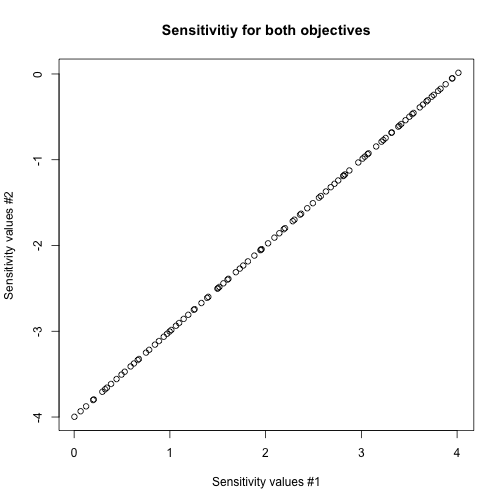Short Description

caRamel is a multiobjective evolutionary algorithm combining the MEAS algorithm and the NGSA-II algorithm.

It is possible to compute the first order derivatives of the Pareto front with caRamel by setting the logical parameter sensitivity to TRUE.

library(caRamel)

Test function

Schaffer

Schaffer test function has two objectives with one variable.

schaffer <- function(i) {
s1 <- x[i,1] * x[i,1]
s2 <- (x[i,1] - 2) * (x[i,1] - 2)
return(c(s1, s2))
}

Note that :

• parameter i is mandatory for the management of parallelism.
• the variable must be named x and is a matrix of size [npopulation, nvariables].

For instance, the variable will lie in the range [-10, 10]:

nvar <- 1 # number of variables
bounds <- matrix(data = 1, nrow = nvar, ncol = 2) # upper and lower bounds
bounds[, 1] <- -10 * bounds[, 1]
bounds[, 2] <- 10 * bounds[, 2]

Both functions are to be minimized:

nobj <- 2 # number of objectives
minmax <- c(FALSE, FALSE) # min and min

Before calling caRamel in order to optimize the Schaffer's problem, some algorithmic parameters need to be set:

popsize <- 100 # size of the genetic population
archsize <- 100 # size of the archive for the Pareto front
maxrun <- 1000 # maximum number of calls
prec <- matrix(1.e-3, nrow = 1, ncol = nobj) # accuracy for the convergence phase

Optimization

Then the minimization problem can be launched with a sensitivity analysis:

results <-
caRamel(nobj,
nvar,
minmax,
bounds,
schaffer,
popsize,
archsize,
maxrun,
prec,
carallel=FALSE,
sensitivity=TRUE) # sensitivity required
## Beginning of caRamel optimization <-- Thu Sep 17 17:05:38 2020
## Number of variables : 1
## Number of functions : 2
## Computing the sensitivity of the Pareto front...
## Done in 4.779865026474 secs --> Thu Sep 17 17:05:43 2020
## Size of the Pareto front : 98
## Number of calls : 1020

Test if the convergence is successful:

print(results\$success==TRUE)
##  TRUE

Plot the Pareto front:

plot(results\$objectives[,1], results\$objectives[,2], main="Schaffer Pareto front", xlab="Objective #1", ylab="Objective #2")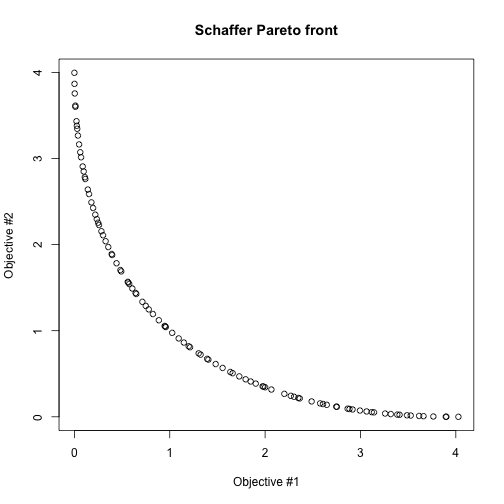plot(results\$parameters, main="Corresponding values for X", xlab="Element of the archive", ylab="X Variable")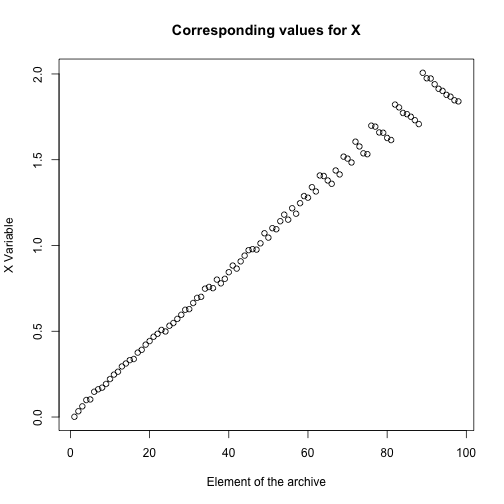Sensitivity

The sensitivity of the Pareto front is evalutated by computing first order derivatives. For each of the objective, one Jacobian matrix is computed:

names(results\$derivatives)
##  "Jacobian_1" "Jacobian_2"

Plot the sensitivity for the first objective:

plot(results\$parameters, results\$derivatives\$Jacobian_1, main="Sensitivitiy for the first objective", ylab="Sensitivity values", xlab="X values")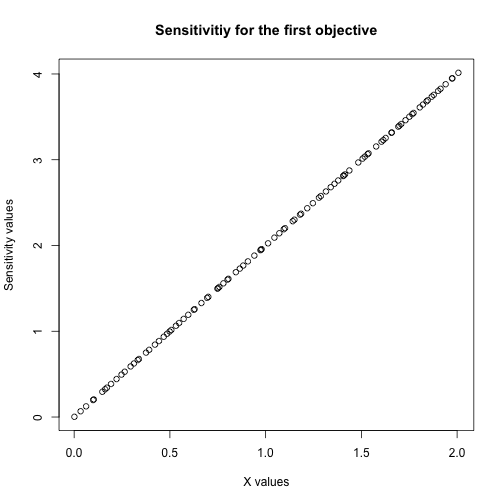Plot the histogram for the second objective:

hist(results\$derivatives\$Jacobian_2, main="Sensitivitiy for the second objective", xlab="Sensitivity values", ylab="Distribution of the Pareto front")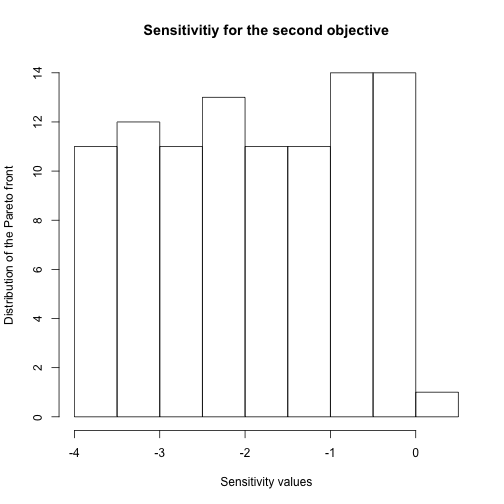Plot the sensitivity of the Pareto front for the two objectives:

plot(results\$derivatives\$Jacobian_1, results\$derivatives\$Jacobian_2, main="Sensitivitiy for both objectives", ylab="Sensitivity values #2", xlab="Sensitivity values #1")